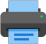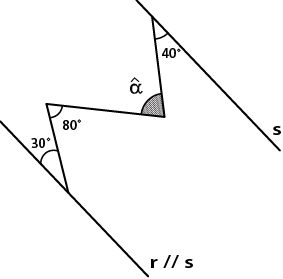Lista de exercícios do ensino médio para impressãoSelect all.
print selected exercises
In the given picture, if r // s , we can say that the value of $\,\hat{\,\alpha\,}\,$ is:a)
90°
b)
100°
c)
110°
d)
120°
e)
22°40'

answer: (E)
×
Knowing that in the picture r // s, the value of $\;\hat{\;x\;}\;$ is:a)
90°
b)
100°
c)
110°
d)
120°
e)
None of the above.

answer: (B)
×
In the picture the line r is parallel to the line s . Find the value of $\,\hat{\,\alpha\,}\,$.answer: α = 90°
×
Other math tests:
Euclidean geometry
angles